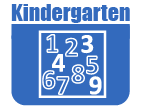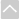# Math - KindergartenReview Activities and Games

These resources are intended for review and practice after initial instruction of the standards.

Strand: MATHEMATICAL PRACTICES (K.MP)Strand: COUNTING AND CARDINALITY (K.CC)
Know number names and the counting sequence (Standards K.CC.1–3).
• Bags of Stuff
The purpose of this task is to give students an opportunity to count real objects and write numbers.
• Choral Counting
The teacher will need a 100 chart or large number line and a pointer. As a whole group, have students chant the counting sequence starting with one to thirty, using the pointer to follow the number sequence. Over time, increase the range to one to fifty and then one to one hundred.
• Counting Circles
This task is a game where students practice counting sequences of no more than 10 numbers.
• Counting by Tens
The objective of this lesson is to gain automaticity counting to 100 and to establish the importance of multiples of ten. The final goal of this lesson is for students to be able to count by tens and articulate the term for this.
This task supports students in correctly writing numbers. Because students have to trace the number instead of coloring in a bubble with the number in it or circling the correct number, they gain handwriting practice as well as counting and addition practice.
• Find The Numbers 0-5 or 5-10
In this task each student places a set of number cards 0-5 face up, in sequence, in front of him or herself. The students take turns rolling the 0-5 die. After rolling he or she needs to find the matching number in the row of cards, say the number name out loud to the other student(s) and turn it face down.
• Five by Two
This game will reinforce the number before and after as well as reading and sequencing numbers.
• Number After Bingo 1-15
This task asks students to play the "Number After" game which resembles bingo and reinforces the student's understanding of the concept of number after.
• Number Line Up
In this activity students are each given a number sign and then must place themselves in number order.
• Number TIC TAC TOE
This activity is a game of tic, tac, toe with students coloring a number on a grid when they hear the number spoken.
• One More Concentration
This game is a version of the traditional memory or concentration game.
• Pick a Number, Counting On
The teacher puts multiple numbers in a hat or on sticks from the known counting sequence. S/he randomly picks one number and asks the class to count on ten numbers from that number. The class does this chorally.
• Race to the Top
This game involves students rolling dice and then writing the number on a grid.
• Rainbow Number Line
In this activity students use crayons to trace numbers on a strip of paper to make their own personal colorful number strip for their desk.
• Start-Stop Counting
This activity is a game similar to Duck, Duck, Goose, with students counting as they touch heads.
• Teen Go Fish
This task is a game using a deck of cards with the numbers 11-19. Students play in small groups with the goal of the game to make pairs of cards with the same number.
Count to tell the number of objects (Standards K.CC. 4–5).
• Color Week
The purpose of this task is to help students understand the connection between counting and cardinality. Thus, oral counting and recording the number in digit form are the most important aspects of this activity.
• Counting Cup
This activity is designed to ensure that students are able to count out their own collection of manipulatives.
• Counting Objects and Ordering Numbers
In this lesson kindergarteners count and order numbers with and without a number line. (6 minutes)
• Finding Equal Groups
The purpose of this task is for students to build fluency in counting.
• IXL Game: Count to 20
This game helps kindergarteners learn how to count to 20. This is just one of many online games that supports the Utah Math core. Note: The IXL site requires subscription for unlimited use.
• Mingle & Count: A Game of Number Sense
This lesson shows a game designed to practice counting by forming groups based on a given number. (5 minutes)
• Number Rods
This activity gives students practice counting for meaning. This task also allows students to see the size of the rod grow as the number gets larger.
Identify and compare quantities of objects and numerals (Standards K.CC.6–7).
• Biggest Number Wins
Given a set of number cards students play a game which requires them to determine which of two numbers is greater.
• Guess the Marbles in the Bag
This activity has students guess how many objects are in a bag and then use the comparison terms less than, greater than or equal to to compare the various guesses.Strand: OPERATIONS AND ALGEBRAIC THINKING (K.OA)
Understand addition as putting together and adding to, and understand subtraction as taking apart and taking from (Standards K.OA.1–5).
This task supports students in correctly writing numbers. Because students have to trace the number instead of coloring in a bubble with the number in it or circling the correct number, they gain handwriting practice as well as counting and addition practice.
This task asks students to write equations, not just record sums, when using dice to generate sums.
• IXL Game: Addition with Pictures - Sums up to 10
This game helps the kindergartener understand addition using objects. This is just one of many online games that supports the Utah Math core. Note: The IXL site requires subscription for unlimited use.
• Make 9
In this task students are asked to make 9 in as many ways as they can by adding two numbers between 0 and 9.
• My Book of Five
The purpose of this task is to help students develop fluency with addition facts to 5.
• Pick Two
This task could be used by teachers as an opportunity to gauge their students' ability to write equations and whether they may be ready to begin a discussion about what the equal sign means.
• Quick Images: Visualizing Number Combinations
The educator in this Teaching Channel video uses quick images to identify combinations of 8 - best done in the latter part of Kindergarten. (6 minutes)
• Shake and Spill
The purpose of this task is for students to decompose a number as a sum of two other numbers in more than one way.
• Ten Flashing Fireflies
The purpose of this task is to help students develop fluency with addition facts to 10.
The purpose of this task is for students to practice adding numbers within ten and become familiar with the ten-frame.
• What's Missing?
For this task the teacher shows the student 6 counters (small, flat objects). The student is then asked to close his/her eyes. while some of the counters are hidden under a sheet of heavy paper. When the student opens his/her eyes, s/he determines how many were hidden based on the number of counters still showing.Strand: NUMBER AND OPERATIONS IN BASE TEN (K.NBT)
Compose and decompose numbers 11–19 to gain foundations for place value (Standard K.NBT.1).Strand: MEASUREMENT AND DATA (K.MD)
Describe and compare measurable attributes of objects (Standards K.MD.1–2)
• Longer and Shorter
The purpose of this task is for students to compare the height of two objects. This task would be good independent practice for students after they have been introduced to the idea of taller and shorter in a whole group setting.
• Which is heavier?
The purpose of this task is for students to use a balance scale to compare the weights of two different objects. It is best if the objects appear to be close to the same weight so that comparing them on a balance scale is meaningful. This task also introduces students to the way in which a balance scale works, which is not obvious.
• Which weighs more? Which weighs less?
In this activity students choose from a varied group of blocks and compare two by weight.
Classify objects and count the number of objects in each category (Standard K.MD.3).
• IXL Game: Sorting, ordering, and classifying: Put numbers up to 30 in order
This game helps the kindergartener classify objects into given categories. This is just one of many online games that supports the Utah Math core. Note: The IXL site requires subscription for unlimited use.
• Sort and Count I
Using cards or various objects students must look at the items and decide two or three different ways to sort them.
• Sort and Count II
The purpose of this task is for students to sort the same set of objects according to different attributes and to practice counting to tell the number of objects in a set.Strand: GEOMETRY (K.G)
Identify and describe shapes, including squares, circles, triangles, rectangles, hexagons, cubes, cones, cylinders, and spheres (Standards K.G.1–3).
• Shape Hunt Part 1
This game is a scavenger hunt. It can be played indoors or outdoors. In the play area, the teacher should scatter 6 shapes cut out of construction paper attached to whatever appropriate surfaces are nearby. The shapes should be clearly numbered. Students go out to the play area with a blank paper with 6 empty slots. When the student finds a shape they should copy it to their own paper in the appropriate spot.
• Shape Hunt Part 2
The main difference between this task and part 1 is that students are now given the task of finding and identifying real-world shapes in their environment.
• Shape Sequence Search
Given a bingo-like sheet showing various shapes the student must find the sequence of shapes that the teacher reads, e.g. triangle-rectangle-rectangle-circle.
Analyze, compare, create, and compose shapes (Standards K.G.4–6).
• Alike or Different Game
In this task/game students are given a set of cards showing various shapes. The student pairs take turns drawing two cards and naming something that is the ALIKE or DIFFERENT between the two cards.
• IXL Game: Identify Shapes
This IXL game helps the kindergartener correctly name shapes. This is just one of many online games that supports the Utah Math core. Note: The IXL site requires subscription for unlimited use.The Online Core Resource pages are a collaborative project between the Utah State Board of Education and the Utah Education Network. If you would like to recommend a high quality resource, contact Trish French (Elementary) or Lindsey Henderson (Secondary). If you find inaccuracies or broken links contact resources@uen.org.